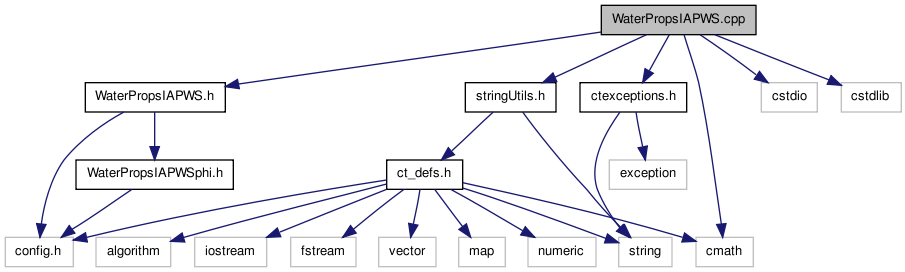# WaterPropsIAPWS.cpp File Reference

Definitions for a class for calculating the equation of state of water from the IAPWS 1995 Formulation based on the steam tables thermodynamic basis (See class WaterPropsIAPWS). More...

`#include "WaterPropsIAPWS.h"`
`#include "ctexceptions.h"`
`#include "stringUtils.h"`
`#include <cmath>`
`#include <cstdio>`
`#include <cstdlib>`
Include dependency graph for WaterPropsIAPWS.cpp:Go to the source code of this file.

## Namespaces

namespace  Cantera

Namespace for the Cantera kernel.

## Defines

#define MAX(x, y)   (( (x) > (y) ) ? (x) : (y))
#define MIN(x, y)   (( (x) < (y) ) ? (x) : (y))

## Variables

const doublereal T_c = 647.096
Critical Temperature value (kelvin).
const doublereal Rho_c = 322.
Value of the Density at the critical point (kg m-3).

## Detailed Description

Definitions for a class for calculating the equation of state of water from the IAPWS 1995 Formulation based on the steam tables thermodynamic basis (See class WaterPropsIAPWS).

Definition in file WaterPropsIAPWS.cpp.

Generated by1.6.3